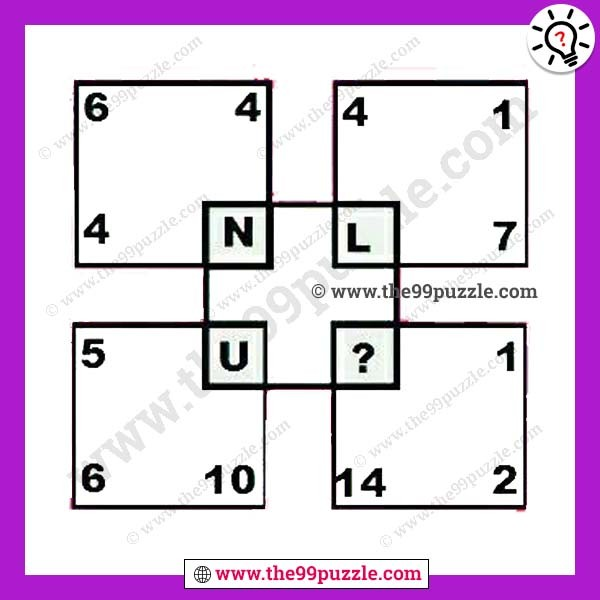# Which letter replaces the question mark? – Puzz140

Which letter replaces the question mark? Can you solve these logical letter puzzles? You can easily improve your logical thinking. Solve the letter puzzles and replaces the question mark.###### Explanation:

Top left box = 4+6+4=14

(A to Z  are respectively assign the numbers 1 to 26)

So, 14 = N

Top right box = 4+1+7=12

(A to Z  are respectively assign the numbers 1 to 26)

So, 12 = L

Bottom left box = 5+6+10=21

(A to Z  are respectively assign the numbers 1 to 26)

So, 21 = U

Bottom right box = 14+2+1=17

(A to Z  are respectively assign the numbers 1 to 26)

So, 17 = Q